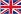(+86)29-6361 0799English

You are here: » » » Square rectangular steel pipe: theoretical weight calculation

# Square rectangular steel pipe: theoretical weight calculation

Views: 1     Author: Site Editor     Publish Time: 2020-11-06      Origin: Site

Carbon steel pipes with square and rectangular (rectangular) cross-sections:

Weight unit per meter: kg/m (kilogram/meter) & lb/ft (lb/ft)

Commonly used square tube calculation formula 1:

(Length + width)×2÷3.14-thickness×thickness×0.02466= kg/m

Formula 2:

Kg/m = (Oc-4Wt) * Wt * 0.00785

Oc is the outer circumference of the steel pipe, Wt is the wall thickness of the steel pipe;

square Oc=4*a

Rectangle Oc=2a+2b a, b is the side length

The popular interpretation is: 4x wall thickness x (side length-wall thickness) x7.85

Among them, the side length and wall thickness of the square tube are in millimeters, and the values are directly substituted into the above formula, and the result is the weight of the square tube per meter, in grams.

Such as a 30x30x2.5 mm square tube, the weight per meter can be calculated according to the above formula:

When the wall thickness and side length are both in mm, 4x wall thickness x (side length-wall thickness) is calculated as the volume of a square tube per meter length, in cubic centimeters, multiplied by the specific gravity of iron per cubic centimeter 7.85 grams is the weight of the square tube per meter in grams.Phone：+86-29-63610799Email：461112563@qq.com